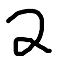# Math Is Fun Forum

Discussion about math, puzzles, games and fun.   Useful symbols: ÷ × ½ √ ∞ ≠ ≤ ≥ ≈ ⇒ ± ∈ Δ θ ∴ ∑ ∫  π  -¹ ² ³ °

You are not logged in.

## #1 2015-11-21 05:10:12

blackcap
Member
Registered: 2015-11-20
Posts: 7

### Explaining primes with a text editor

Suppose you have a number n, and that you write a list of n modulo x, where x is all numbers between n and 1. Do this for all integers greater than 0.

``````1: 0
2: 0 0
3: 0 1 0
4: 0 1 0 0
...``````

1 is divisible by 1.
2 is divisible by 1 and 2.
3 is divisible by 1 and 3, and has a remainder of 1 when divided by 2.
4 is divisible by 1, 2 and 4, and has a remainder of 1 when divided by 3.
..

Notice that the modulos are in the reverse order, such that the first number on a line is n modulo n, and the next is n modulo (n-1).

This is a screenshot of a text editor with the pattern for the first 300 numbers or so, with 0 highlighted.You get a bunch of lines! The leftmost line is the n%n line. All numbers are divisible by themselves. The next line is n%(n/2), all numbers that are divisible by 2, are also divisible by themselves divided by two. The pattern continues like this, the next line being n%(n/3).

``````..
18: 000 001 002 003 004 005 006 007 008 000 002 004 000 003 002 000 000 000
19: 000 001 002 003 004 005 006 007 008 009 001 003 005 001 004 003 001 001 000
20: 000 001 002 003 004 005 006 007 008 009 000 002 004 006 002 000 000 002 000 000
21: 000 001 002 003 004 005 006 007 008 009 010 001 003 005 000 003 001 001 000 001 000
..``````

Notice that between the 1 and 2 line, the modulos are incrementing by one up to ceiling(n/2-1). After the 2 line, everything increments by 2.
You also see a example of diagonal incrementation lines, that is, you can pick any of the modulo numbers, go one down and one to the right, and that number is going to be one greater than the number that you came from, unless it is zero.Consider 6:

``````06: 000 001 002 000 000 000
07:                         000
08:                         000
09:                         000
10:                         002
11:                         001
12:                         000
13:                         006
14:                         006
15:                         006
...``````

If you flip this sequence 90 degrees, it is going to replicate:
6 is divisible by 1, therefor 7 is divisible by 1.
6 is divisible by 2, therefor 8 is divisible by 2.
6 is divisible by 3, therefor 9 is divisible by 3.
6 has a remainder of 2 when divided by 4, therefor 10 has a remainder of 2 when divided by 4.
6 has a remainder of 1 when divided by 5, therefor 11 has a remainder of 1 when divided by 5.
6 is divisible by 6, therefor 12 is divisible by 6.

after this point, 6 is just going to repeat infinitely downwards. This pattern is true for all numbers, and primes are just the unlucky numbers that didn't get any zeroes.
I am sure that there are a lot more patterns that I didn't cover, so I'm gonna leave that to the comment sectionHaskell program that generates the first 1000 lines in case anyone want it:

``````mods x = map (mod x) [1..x]
mods'  = map  mods [1..]

show' x = replicate 3 - length (show x) '0' ++ show x

main = putStrLn . unlines . map (unwords.map show'.reverse) \$ take 1000 mods'``````

Last edited by blackcap (2015-11-21 06:39:25)

Offline

## #2 2016-02-12 02:04:53

mathaholic
MemberFrom: Earth
Registered: 2012-11-29
Posts: 3,251

### Re: Explaining primes with a text editor

I see.